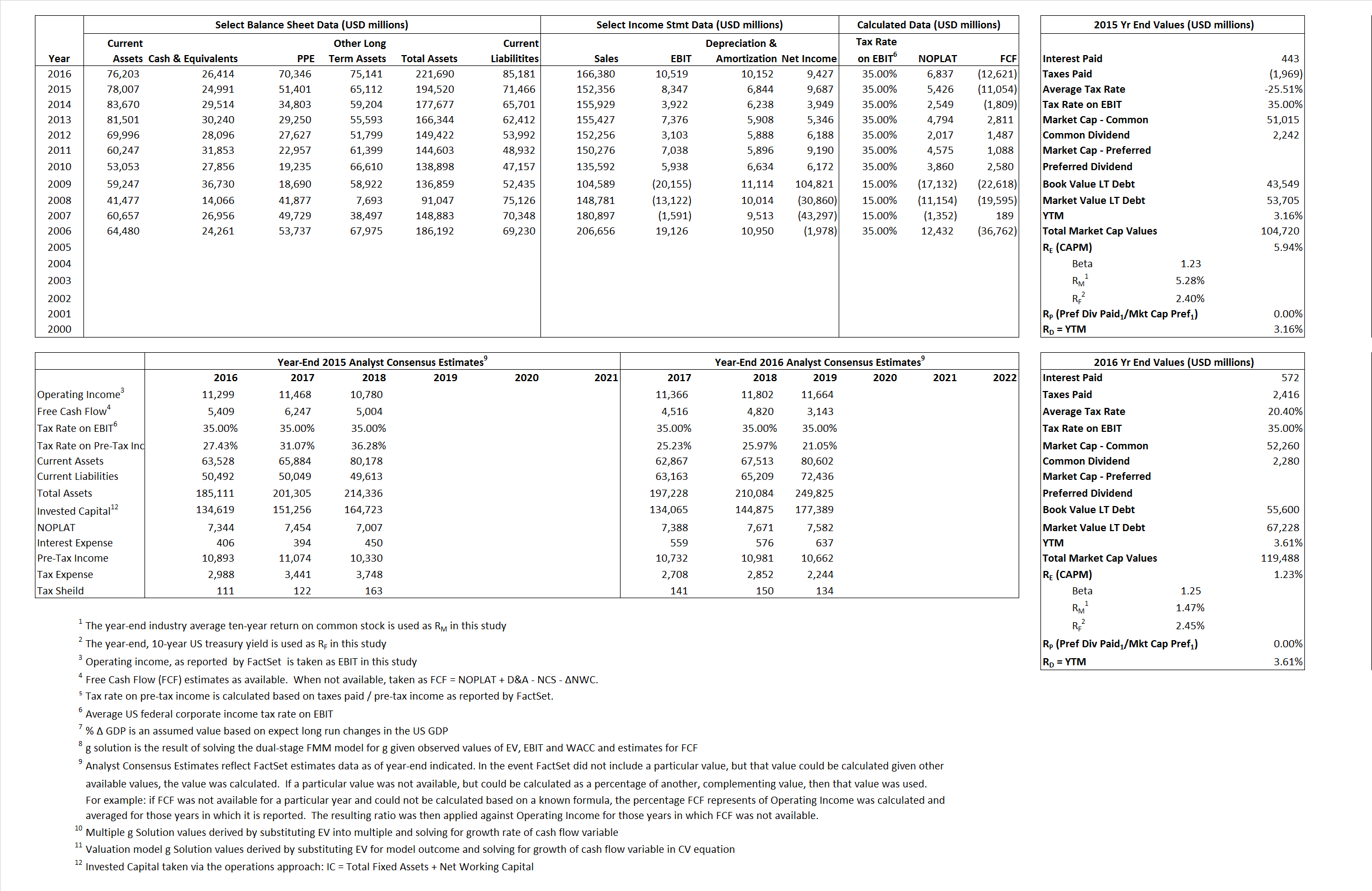# General Motors Company

## Analyst Listing

The following analysts provide coverage for the subject firm as of May 2016:

 Broker Analyst Analyst Email Buckingham Research Joseph C. Amaturo jamaturo@buckresearch.com Susquehanna Financial Group Matthew T. Stover matthew.stover@sig.com Deutsche Bank Research Rod Lache rod.lache@db.com Evercore ISI George Galliers george.galliers@evercoreisi.com RBC Capital Markets Joseph Spak joseph.spak@rbccm.com CRT Capital Group Michael Ward mward@sterneageecrt.com Craig Hallum Capital Group Steve L. Dyer sdyer@craig-hallum.com Daiwa Securities Co. Ltd. Jairam Nathan jairam.nathan@us.daiwacm.com

## Primary Input Data## Derived Input Data

### Equational Form

Net Operating Profit Less Adjusted Taxes NOPLAT 5,426  6,837$NOPLAT\, =\, EBIT\, x\, (1 \,-\, Avg \,\,Tax\,\, Rate\,\, on\,\, EBIT)$
Free Cash Flow FCF (11,054) (12,621)$FCF\,=NOPLAT\,+\,Non-Cash\,Expenses-\Delta NWC\,-\,NCS$
Tax Shield TS (113) 117$TS\,=\,Interest\,\,Paid\,\,x\,\, Avg \,\,Tax\,\,Rate\,\, on\,\, Pre-Tax\,\, Income$
Invested Capital IC 123,054 136,509$IC\,=\,Fixed\,\,Operating\,\,Assets\,\,+\,\,Net\,\, Working\,\, Capital$
Return on Invested Capital ROIC 4.41% 5.01%$ROIC\,=\,\frac { NOPLAT }{ IC }$
Net Investment NetInv  17,922 23,607$NetInv\,=\,{ {IC}_{1}}-{{IC}_{0}}+Depreciation$
Investment Rate IR  330.33% 345.27%$IR\,=\,\frac {NetInv}{NOPLAT}$
Weighted Average Cost of Capital
WACCMarket  4.93% 2.15%$WACC\,=\,\frac { E }{ V } { R }_{ E }\,+\,\frac { P }{ V } { R }_{ P }\,+\,\frac { D }{ V } { R }_{ D }\left( 1- Avg\,\, Tax\,\,Rate\,\,on\,\,Pre-Tax\,\,Income \right)$
WACCBook    6.55%   5.52%
Enterprise value
EVMarket  79,729 93,074$EV\,=\,Market\,\,Cap\,\,Equity\,+\,\,Long\,\,Term\,\,Debt\,-\,Cash$
EVBook    69,573  81,443
Long-Run Growth
g = IR x ROIC
14.56%   17.29% Long-run growth rates of the income variable are used in the Continuing Value portion of the valuation models.
g = %$\Delta$ GDP    2.50%    2.50%
Margin from Operations M   5.48%   6.32%$M\,\,=\,\,\frac{EBIT}{SALES}$
Depreciation/Amortization Rate D   45.05%   49.11%$D\,\,=\,\,\frac{D+A}{EBITDA}$

## Valuation Multiple Outcomes

The outcomes presented in this study are the result of original input data, derived data, and synthesized inputs.

### model g solution

12/31/2015 12/31/2016 12/31/2015 12/31/2016 12/31/2015 12/31/2016

EV/SALES$\frac {EV}{Sales} \,= \,\frac{ROIC\, -\, g}{ROIC\,(WACC\,-\,g)}\,(1\,-\,T)\,(M)$

0.52  o.56  3.45%  11.13%  3.61%  12.61%

EV/EBITDA$\frac {EV}{EBITDA} \,= \,\frac{ROIC\, -\, g}{ROIC\,(WACC\,-\,g)}\,(1\,-\,T)\,(1\,-\,D)$

5.25 4.50 3.45% 11.13% 3.61% 12.61%

EV/NOPLAT$\frac {EV}{NOPLAT} \,= \,\frac{ROIC\, -\, g}{ROIC\,(WACC\,-\,g)}$

14.70 13.61 3.45% 11.13% 3.61% 12.61%

EV/FCFOPS$\frac {EV}{FCF_{OPS}} \,= \,\frac{ROIC\, -\, g}{ROIC\,(WACC\,-\,g)}\,(1\,-\,T)$

-7.21 -7.37 3.45% 11.13% 3.61% 12.61%

EV/EBIT$\frac {EV}{EBIT} \,= \,\frac{ROIC\, -\, g}{ROIC\,(WACC\,-\,g)}\,(1\,-\,T)$

9.55 8.85 3.45% 11.13% 3.61% 12.61%

EV/IC$\frac {EV}{IC} \,= \,\frac{ROIC\, -\, g}{WACC\,-\,g}$

0.65 0.68 3.45% 11.13% 3.61% 12.61%